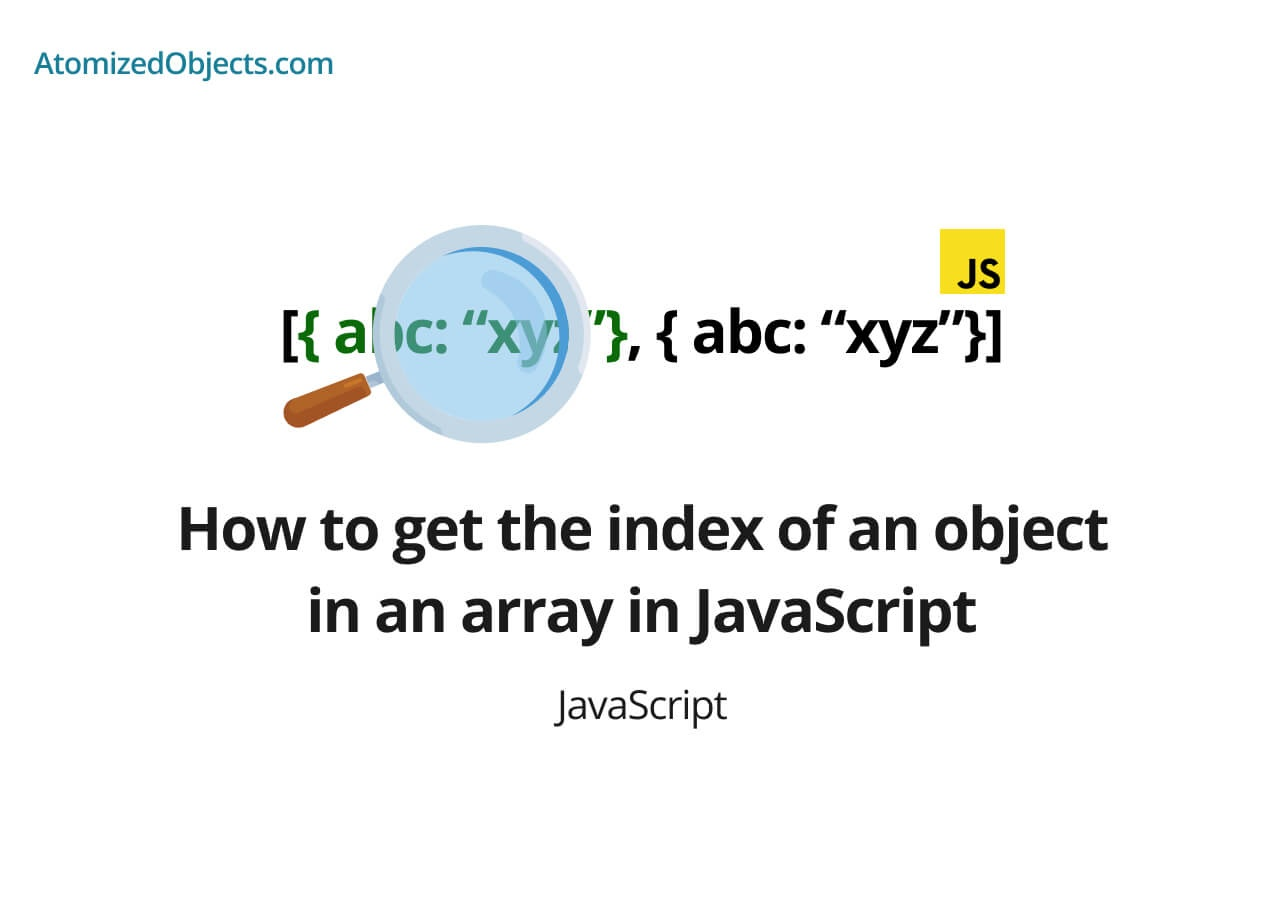Blog / Javascript

# How to get the index of an object in an array in JavaScriptFind out how to get the index of an object in an array in JavaScript using findIndex and indexOf as well as how referential equality effects it.Will Mayger
August 24, 2021
Article

Finding the index of an object in an array in JavaScript is a little different from finding the index of a string or a number because when you compare objects in JavaScript you need to keep in mind something called referential equality.

In this post we will cover how to get the index of an object in an array in JavaScript, what referential equality is and how to get the index of an object in an array in JavaScript if you do have referential equality.

A quick note before we get started, if you want a more in-depth guide to How to get the index of an item in an array in JavaScript using indexOf, I have just finished writing a post here all about it.

Let’s get started.

## How to get the index of an object in an array in JavaScript

To get the index of an object in an array in javascript we need to use the Array.prototype.findIndex method in order to be able to look at object properties, keys or values which we can then use to perform our comparisons to find the index of the object we are looking for.

The findIndex method is not quite as commonly known as the Array.prototype.indexOf method but it does a similar thing, just using a callback instead so we can provide our own comparison function.

This is needed because of something called referential equality.

Referential equality means that when we are comparing two objects, unless they refer to the exact same object then the comparison will fail.

All this means is two identical objects do not equal one another in JavaScript unless they are the same object.

Here is an example of this:

``````{} === {} // false

const obj = {}
obj === obj // true``````

Now that we know how why we need the callback, let’s take a look at how to get the index of an object in an array in JavaScript using Array.prototype.findIndex:

``````const myArray = [{ apples: 10 }, { apples: 15 }, { apples: 4 }]

myArray.findIndex(arrayItem => arrayItem.apples === 15) // 1
myArray.findIndex(arrayItem => arrayItem.apples === 10) // 0
myArray.findIndex(arrayItem => arrayItem.apples === 4) // 2
myArray.findIndex(arrayItem => arrayItem.apples === 5) // -1``````

As you can see in the above example of how to get the index of an object in an array in JavaScript using the findIndex method all we need to do is pass in a function into findIndex that returns a boolean.

If the boolean we return is true then findIndex knows that is the correct index and it will return the index to us as a number, if it returns false then it knows it needs to keep looking until it goes through each item in the array.

If the findIndex method cant find the item, it will return -1 just like when using `indexOf`.

So to get or find the index of an object in an array in JavaScript you can use the `findIndex` method with a callback that returns a boolean of true or false when provided with the correct item.

This can be done with any property, key or value in the objects within your array.

### How to get the index of an object in an array in JavaScript with referential equality

You can use Array.prototype.indexOf in certain circumstances, but only when you know you have referential equality.

Here is an example:

``````const myObj = { apples: 15 }
const myArray = [{ apples: 10 }, myObj, { apples: 4 }]

myArray.indexOf(myObj) // 1``````

This only works because the object in the array is referencially equal with the one we are looking for meaning that both the one in the array and the one we are using for the comparison are pointing to the same reference.

If we try this with identical objects that are not referencially equal instead it won’t be able to find the correct object and will instead return `-1`.

Here is an example of this:

``````const myArray = [{ apples: 10 }, { apples: 15 }, { apples: 4 }]
myArray.indexOf({ apples: 15 }) // -1``````

If you are in doubt of which one to use, it is best to stick with findIndex to look inside of each object for properties because it will be more versatile and give you results closer to how you expect than indexOf will.

### Summary

There we have how to get the index of an object in an array in JavaScript, if you want more like this be sure to check out some of my other posts!Foxi

Budget Planner & Tracker

More money in your pocket by the end of the month.

Free to use and no account needed.

Get started now.

Some graphics used on this post were made using icons from flaticon.